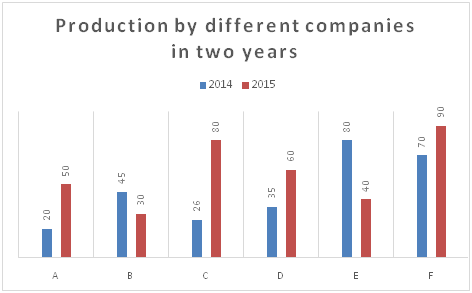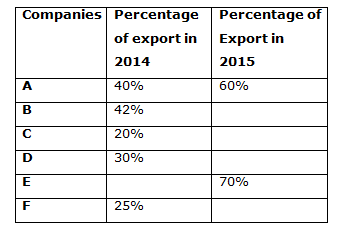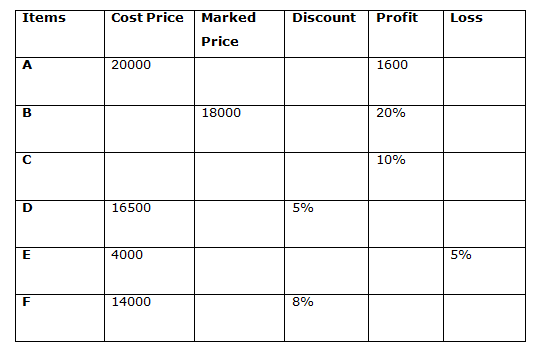# Quantitative Aptitude Questions (Data Interpretation) for RRB PO Mains 2018 Day-183

Dear Readers, IBPS RRB is conducting Online Examination for the recruitment of probationary officer. To enrich your preparation here we have providing new series of Data Interpretation – Quantitative Aptitude Questions. Candidates those who are appearing in RRB PO Mains Exams can practice these Quantitative Aptitude average questions daily and make your preparation effective.

[WpProQuiz 3301]

Click “Start Quiz” to attend these Questions and view Solutions

Directions (1- 5): Study the following graph carefully and answer the given questions:

The graph below show production of mobiles (in thousands) units by different companies in two different years:1) The average number of mobiles exported by company D and F in 2015 is 35000 while the mobiles exported by company D in 2014 is twice the export in 2015 then find the approximate percentage of export by company F out of total production of company F in 2015?

a) 60%

b) 72%

c) 84%

d) 55%

e) 90%

2) If company E exported a total of 50000 mobiles in the year 2014 and 2015 and the ratio between the export in 2014 and 2016 is 11: 5 then find the number of mobiles produced by company E in 2016 if export is 80% of the production?

a) 11120

b) 12500

c) 19890

d) 10250

e) 12750

3) The total number of mobiles exported by company A in 2014 and 2015 is 2/3 of the mobiles exported by company C in these years then find the percentage of mobiles that were exported by company C out of total production in 2015 ?

a) 90.75%

b) 67.5%

c) 25.75%

d) 64.75%

e) 56.25%

4) The 20% of the mobiles exported by company B in 2014 are defective while the ratio between the defective mobiles exported by company B in the year 2014 and 2015 is 7 : 5 and the percentage of defective mobiles out of total export by company B in 2015 is 13.5% then find the approximate percentage of mobile exported by company B out of total production?

a) 45 %

b) 70 %

c) 55 %

d) 67 %

e) 35 %

5) The mobiles exported by company A, B and D in 2014 is approximately what percent the export by A and E in 2015?

a) 45%

b) 57%

c) 65%

d) 76%

e) 56%

Directions (6 – 10): Study the following table carefully and answer the given questions:

The table below shows data related to sales of different items.6) If the shopkeeper marked up the price of the item A by 20% and due to less demand the shopkeeper allowed x % discount on the item then find the value of x?

a) 25

b) 21

c) 10

d) 60

e) 56

7) The shopkeeper allowed two successive discount of 10% and 20% on the item B and the shopkeeper paid 5% commission on selling price to an agent for selling the item, then find the profit percentage if he would have allowed a single discount of 5% and no commission for selling the item?

a) 25.66 %

b) 21.88 %

c) 19.66 %

d) 66.66 %

e) 56.66 %

8) If the shopkeeper had bought the item C at 4% less and sold it for Rs 60 more he would have gained (75/4)% then find the cost price of the item C?

a) 25000

b) 15000

c) 19000

d) 6000

e) 5600

9) If the difference between the selling price of the Item E when 10% and 20% discount is allowed is Rs 432 then find by how much percentage should the shopkeeper allow discount so that he neither gain or loss by selling the item?

a) 2.5

b) 2.1

c) 7.4

d) 6.7

e) 5.6

10) If the market price of item D and F are equal and the selling price of item D is Rs 801 more than selling price of item F then find the approximate profit or loss percentage earned/incurred by the shopkeeper by selling the item D and F together?

a) 25

b) 21

c) 19

d) 64

e) 56

Total mobiles exported by company D and F= 35000*2= 70000

Mobile exported by company D in 2014= 30/100* 35000= 10500

Mobile exported by company D in 2015= 10500/2= 5250

So,

Mobile exported by company F= 70000- 5250= 64750

Percentage of production exported by company F in 2015

= > (64750/90000)*100 = 72%

Mobile exported by company E in 2015 = 40000*(70/100) = 28000

Mobile exported by company E in 2014 = 50000- 28000 = 22000

Mobile exported by company E in 2016 = (22000/11)*5 = 10000

Number of mobiles produced by company E = (10000/80)*100 = 12500

Total number of mobiles exported by company A in 2014 and 2015

= 40/100* 20000 + 60/100* 50000

= 8000 + 30000

= 38000

Mobile exported by company C in 2014 and 2015= 38000 *3/2= 57000

Mobile exported by company C in 2015

= 57000 – (26000* 20/100)

= 51800

So,

Percentage of export by company C

= (51800/80000)*100 = 64.75 %

Number of defective mobile exported by B in 2014

= 45000*42/100* 20/100=3780

Number of defective mobile exported by company B in 2015= 3780/7* 5= 2700

Number of mobiles exported by company B= 2700/13.5 * 100= 20000

So,

Percentage of mobile exported by company B in 2015

= > (20000*100)/30000 = 66.66 % = 67 %

Mobile exported by A, B and D in 2014

= 40*20000/100 + 42* 45000/100 + 30* 35000/100

= 8000 + 18900 + 10500

= 37400

Mobile exported by company A and E in 2015

= 50000*60/100 + 70*40000/100

= 30000 + 28000

= 58000

Required percentage = (37400*100)/58000 = 65%

Marked up price of item A= 20000* 120/100= 24000

So,

Selling price= 24000-24000*x/100

24000-240x– 20000= 1600

4000- 240x= 1600

240x= 4000-1600

x= 2400/240

x= 10%

Selling price of item B=18000*90/100*80/100= Rs 12960

Commission paid to agent= 12960*5/100= Rs 648

Selling price of the item after giving commission = 12960-648= Rs 12312

Cost price of item B= 12312*100/120= Rs 10260

Selling price when one single discount is allowed= 18000*95/100= Rs 17100

Profit earned= 17100- 10260= Rs 6840

Profit percentage= 6840*100/10260= 66.66%

Let the cost price of the item C be x

Selling price = x*110/100= 11x/10

New cost price= x*96/100= 96x/100= 24x/25

New selling price= 11x/10 + 60

So,

(11x+600)/10 = 475/400 *24x/25

(11x + 600)/10 = 57x/50

550x + 30000 = 570x

30000 = 20x

X = Rs 15000

Let the marked price of item E be x

So,

x *90/100 – x*80/100= 432

90x/100 – 80x/100= 432

10x/100= 432

x= 432*100/10

x= Rs 4320

Discount to be allowed = 4320-4000 = Rs 320

Discount percentage= 320*100/4320= 7.4%

Let the marked price of item D and F be x

Selling price of item D= x*95/100

Selling price of item F= x*92/100

So,

95x- 92x/100 = 801

3x= 801*100

x= 801*100/3

x= Rs 26700

Selling price of D= 26700*95/100= Rs 25365

Profit in item D= 25365- 16500= Rs 8865

Selling price of item F= 26700*92/100= Rs 24564

Profit in item F= 24564- 14000= Rs 10564

Total profit earned in D and F= 10564+8865= 19429

Profit percentage= 19429*100/ 30500= 63.70 %

Daily Practice Test Schedule | Good Luck

 Topic Daily Publishing Time Daily News Papers & Editorials 8.00 AM Current Affairs Quiz 9.00 AM Quantitative Aptitude “20-20” 11.00 AM Vocabulary (Based on The Hindu) 12.00 PM General Awareness “20-20” 1.00 PM English Language “20-20” 2.00 PM Reasoning Puzzles & Seating 4.00 PM Daily Current Affairs Updates 5.00 PM Data Interpretation / Application Sums (Topic Wise) 6.00 PM Reasoning Ability “20-20” 7.00 PM English Language (New Pattern Questions) 8.00 PM